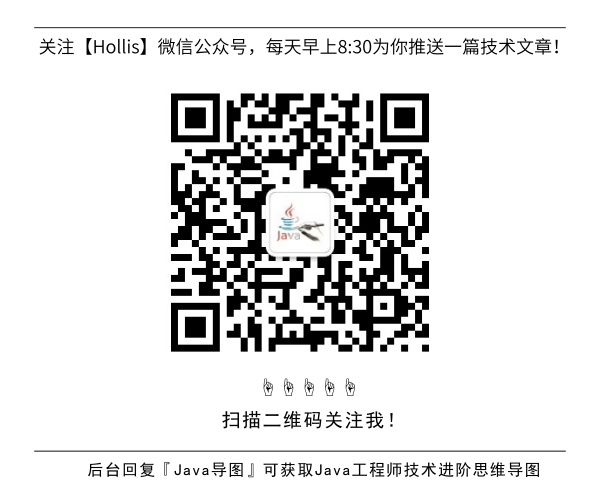# 单元测试第二弹——单元测试与单元测试框架

GitHub 19k Star 的Java工程师成神之路，不来了解一下吗！

## 对代码做白盒测试

• 初始化测试环境、准备测试数据。
• 调用需要被测试的单元。
• 收集结果，并与期望值比较。
• 测试数据清理。

``````public class Calculator{
public float divide(float divisor，float dividend){
return divisor/dividend;
}
}
``````

``````public class CalculatorTest{
public static void main(String [] args){
Calculator calculator = new Calculator();
float result = calculator. divide(10.0,2.0);
if(result == 5.0){
System.out.println("divide test ok");
}else{
System.out.println("divide test failed");
}
}
}
``````

## 单元测试框架

• 测试工具

• 测试工具是一整套固定的工具用于基线测试。测试工具的目的是为了确保测试能够在共享且固定的环境中运行，因此保证测试结果的可重复性。一般负责初始化测试环境、准备测试数据和测试数据清理。
• 测试套件

• 测试套件意味捆绑几个测试案例并且同时运行。
• 测试运行器

• 用于执行测试案例。一般负责调用需要被测试的单元、收集结果、并与期望值比较。

## 参考资料

【软件测试】 黑盒测试、白盒测试、灰盒测试

(全文完)## HollisChuang's Blog

• 微信咨询
• 去评论
• 回顶
###### 回顶部